# Understanding for-loop in Python

• Last Updated : 29 Dec, 2019

A Pythonic for-loop is very different from for-loops of other programming language. A for-loop in Python is used to loop over an iterator however in other languages, it is used to loop over a condition. In this article, we will take a deeper dive into Pythonic for-loop and witness the reason behind this dissimilarity. Let’s begin by familiarizing ourselves with the looping gotchas:

## Gotchas in for-loop

If one doesn’t know what “gotcha” means: a “gotcha” in coding is a term used for a feature of a programming language (say for-loop, function, return statement, etc) that is likely to play tricks by showing a behavior which doesn’t match the expected outcome. Here are two infamous for-loop gotchas:

Consider this example:

 `numList ``=` `[``0``, ``1``, ``2``, ``3``, ``4``]``squares ``=` `(n``*``*``2` `for` `n ``in` `numList)`

Here, the variable `squares` contains an iterable of squares of the elements of `numList`. If we check whether 16 is in `squares`, we get True but if we check it again, we get False.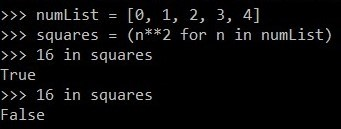sum of a for-loop :

Take a look at this:

 `numList ``=` `[``0``, ``2``, ``4``, ``6``, ``8``]``doubles ``=` `(n ``*` `2` `for` `n ``in` `numList)`

We can make this `doubles` into a list or tuple to look at its elements. Let’s calculate the sum of the elements in doubles. The result should be 40 as per expectation.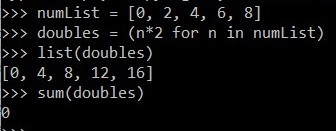To understand this anomaly, let’s first see “under-the-hood” working of for-loops.

## Inside a for-loop

As stated earlier, for-loops of other programming languages, such as C, C++, Java, loop over a condition. For example:

 `let numList = [0, 1, 2, 3, 4];``for` `(let i = 0; i < numList.length; i += 1) {``    ``print(numList[i])``}`

The above code is written in Javascript. As seen the for-loop is quite different from what we see in Python. This is because what we call a for-loop in Python is actually a “foreach” loop. A foreach loop doesn’t maintain a counter like a for loop. A for loop works on indices of the elements of the iterable rather than the element itself. But a foreach loop works straight on the elements rather than their indices and thus have no conditions, no initializations and no incrementation of index.

 `numList ``=` `[``0``, ``1``, ``2``, ``3``, ``4``]`` ` `# for loop in Python``for` `n ``in` `numList: ``    ``print``(n)`

Hence, it won’t be wrong to say that we don’t have for-loops in Python but we have foreach loops which are implemented as for-loops!
One might think that Python uses indices under the hood to loop over in a for loop. But the answer is no. Let’s look at an example to prove this:
We will take the help of while loop for using indices.

 `games = { ``'tennis'``, ``'baseball'``, ``'rugby'``, ``'soccer'` `} ``i = 0`` ` `while` `i < len(games):``    ``print(games[i])``    ``i += 1`

Output: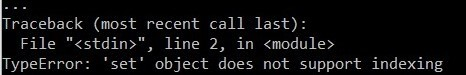This proves that Python doesn’t make use of indices for looping and so we can’t loop over everything using indices. A simple question now arises, what does Python use for looping? The answer is, iterators!

## Iterators

We know what iterables are(lists, strings, tuples, etc). An iterator can be considered as the power supply of iterables. An iterable is made up of iterator and this is the fact which helps Python to loop over an iterable. To extractor iterator from an iterable, we use Python’s `iter` function.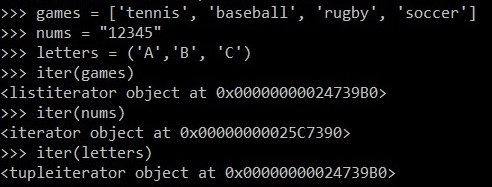Let’s look at an example:

 `games ``=` `[``'tennis'``, ``'baseball'``, ``'rugby'``, ``'soccer'``]``iterator ``=` `iter``(games)`` ` `# we use the next() function to ``# print the next item of iterable``print``(``next``(iterator)) ``print``(``next``(iterator))``print``(``next``(iterator))`

If we keep on using the `next()` function even after we reach the last item, we will get a StopIteration Error.

Note: Once an item in an iterator is all used up(iterated over), it is deleted from the memory!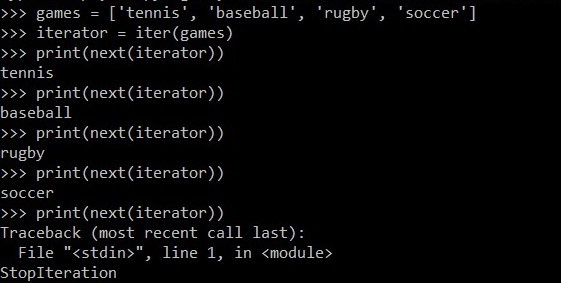Now that we know how loops work, let’s try and make our own loop using the power of iterators.

 `# Python program to demonstrate``# power of iterators`` ` ` ` `# creating our own loop``def` `newForLoop(iterable): `` ` `    ``# extracting iterator out of iterable``    ``iterator ``=` `iter``(iterable) `` ` `    ``# condition to check if looping is done``    ``loopingFinished ``=` `False` ` ` `    ``while` `not` `loopingFinished:``        ``try``:``            ``nextItem ``=` `next``(iterator)``        ``except` `StopIteration:``            ``loopingFinished ``=` `True``        ``else``:``            ``print``(nextItem)``             ` `# Driver's code``newForLoop([``1``, ``2``, ``3``, ``4``])`

Output:

```1
2
3
4
```

We need to learn about iterators because we work with iterators almost every time with even knowing about it. The most common example is a generator. A generator is an iterator. We can apply each and every function of an iterator to a generator.

 `numList ``=` `[``0``, ``2``, ``4``]`` ` `# creating a generator named "squares"``squares ``=` `(n``*``*``2` `for` `n ``in` `numList) `` ` `print``(``next``(squares))``print``(``next``(squares))``print``(``next``(squares))`

Output:

```0
4
16
```

## Resolving looping gotchas

Now that we know what exactly are for-loops and how do they work in Python, we will end this article from where we began, that is, by trying to reason out looping gotchas as seen earlier.

Exhausting an iterator partially :

When we did :

 `numList ``=` `[``0``, ``1``, ``2``, ``3``, ``4``]``squares ``=` `(n``*``*``2` `for` `n ``in` `numList)`

and asked if 9 is in the `squares`, we got True. But asking again gives returns a False. This is because when we first asked if 9 is there, it iterates over the iterator(generator) to find 9 and we know that as soon as the next item in an iterator is reached, the previous item is deleted. This is why once we find 9, all the numbers before 9 get deleted and asking again returns False. Hence we have exhausted the iterator partially.

Exhausting an iterator completely :

In this code:

 `numList ``=` `[``0``, ``2``, ``4``, ``6``, ``8``]``doubles ``=` `(n ``*` `2` `for` `n ``in` `numList)`

When we convert `doubles` to list, we are already iterating over each item in the iterator by doing so. Therefore, the iterator gets completely exhausted and finally, no items remain in it. This is why the function `sum()` on the tuple returns zero. If we do the sum without converting it into a list, it will return the correct output.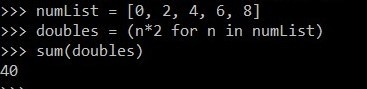My Personal Notes arrow_drop_up